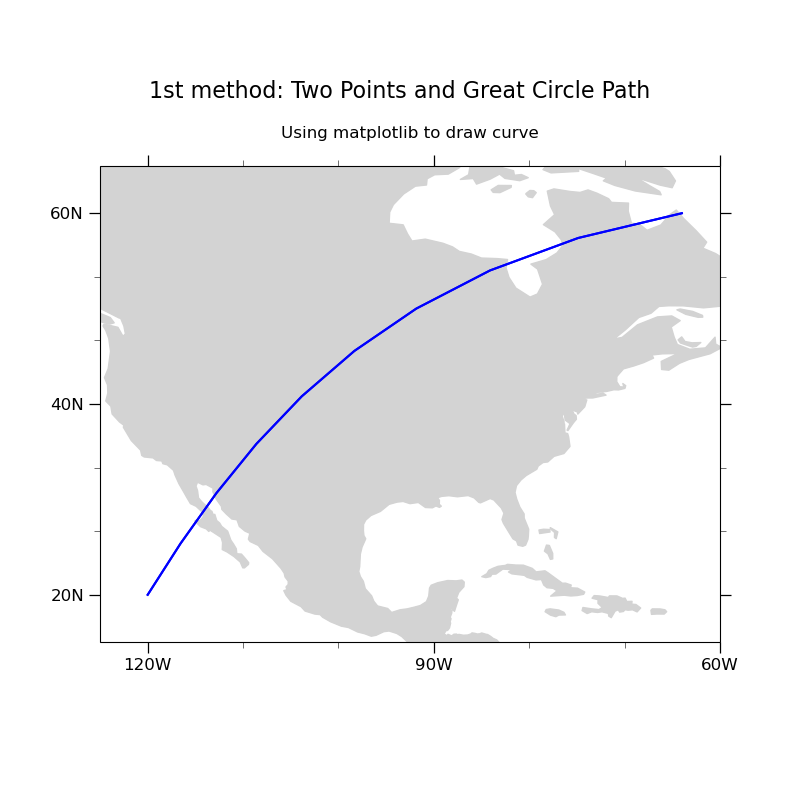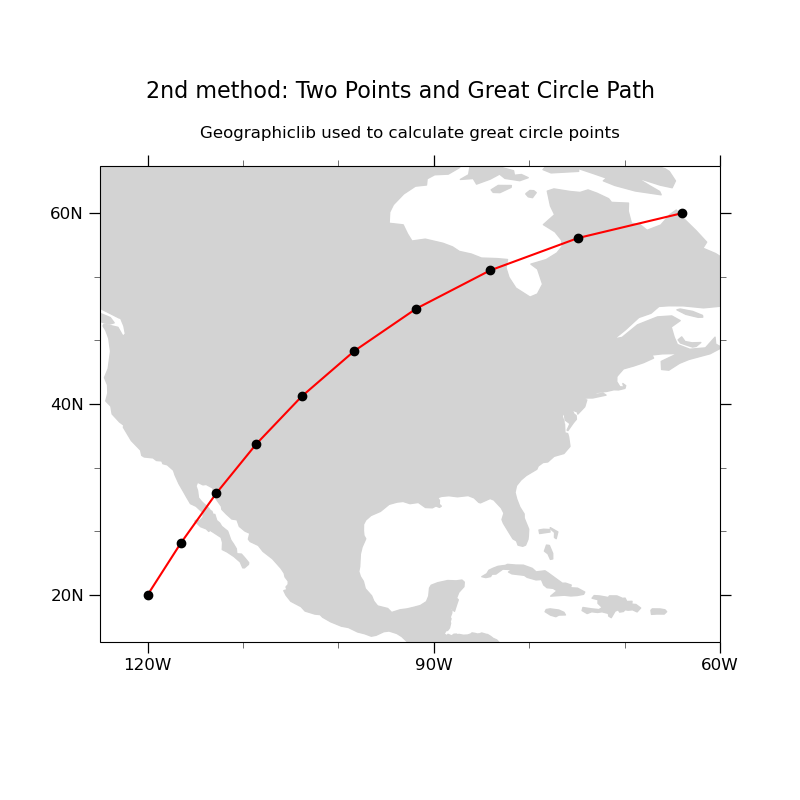# NCL_polyg_14.py#

This script illustrates the following concepts:
• Drawing polylines and markers using great circle paths

• Using geographiclib to calculate a great circle path

• Attaching polylines and markers to a map plot

See following URLs to see the reproduced NCL plot & script:
```# Import packages:

import numpy as np
import cartopy.crs as ccrs
import matplotlib.pyplot as plt
import cartopy.feature as cfeature
from geographiclib.geodesic import Geodesic
from cartopy.mpl.gridliner import LongitudeFormatter, LatitudeFormatter

import geocat.viz as gv
```

Plot

```def Plot(color, ext, xext, yext, npts, title, subt, style, pt):
"""Helper function to create two similar plots where color, extent, title,
line style, and marker style can all be customized on the same style map
projection.

Args:

color (:class: 'str'):
color for line on map in format 'color'
ext (:class: 'list'):
extent of the projection view in format [minlon, maxlon, minlat, maxlat]
xext (:class: 'list'):
start and stop points for curve in format [startlon, stoplon]
yext (:class: 'list'):
start and stop points for curve in format [startlat, stoplat]
title (:class: 'str'):
title of graph in format "Title"
style (:class: 'str'):
line style in format 'style'
pt (:class: 'str'):
marker type in format 'type'
"""
plt.figure(figsize=(8, 8))
ax = plt.axes(projection=ccrs.PlateCarree())

ax.set_extent(ext, ccrs.PlateCarree())

# This gets geodesic between the two points
# WGS84 ellipsoid is used
# yext and xext refer to the start and stop points for the curve
#  being start,  being stop
gl = Geodesic.WGS84.InverseLine(yext, xext, yext, xext)
npoints = npts

# Compute points on the geodesic, and plot them
# gl.s13 is the total length of the geodesic
# the points are equally spaced by 'true distance', but visually
# there is a slight distortion due to curvature/projection style
lons = []
lats = []
for ea in np.linspace(0, gl.s13, npoints):
g = gl.Position(ea, Geodesic.STANDARD | Geodesic.LONG_UNROLL)
lon2 = g["lon2"]
lat2 = g["lat2"]
lons.append(lon2)
lats.append(lat2)

plt.plot(lons, lats, style, color=color, transform=ccrs.Geodetic())
ax.plot(lons, lats, pt, transform=ccrs.PlateCarree())

plt.suptitle(title, y=0.90, fontsize=16)

# Use geocat.viz.util convenience function to set axes parameters without calling several matplotlib functions
# Set axes limits, and tick values
gv.set_axes_limits_and_ticks(
ax,
xlim=(-125, -60),
ylim=(15, 65),
xticks=np.linspace(-180, 180, 13),
yticks=np.linspace(0, 80, 5),
)

# Use geocat.viz.util convenience function to make plots look like NCL plots by using latitude, longitude tick labels

# Remove the degree symbol from tick labels
ax.yaxis.set_major_formatter(LatitudeFormatter(degree_symbol=""))
ax.xaxis.set_major_formatter(LongitudeFormatter(degree_symbol=""))

# Use geocat.viz.util convenience function to add minor and major tick lines

# Use geocat.viz.util convenience function to set titles and labels without calling several matplotlib functions
gv.set_titles_and_labels(ax,
maintitle=subt,
maintitlefontsize=12,
xlabel="",
ylabel="")
plt.show()

# plot first color map
Plot(
"blue",
[-125, -60, 15, 65],
[-120, -64],
[20, 60],
10,
"1st method: Two Points and Great Circle Path",
"Using matplotlib to draw curve",
"-",
"blue",
)

# plot second color map
Plot(
"red",
[-125, -60, 15, 65],
[-120, -64],
[20, 60],
10,
"2nd method: Two Points and Great Circle Path",
"Geographiclib used to calculate great circle points",
"-",
"ko",
)
```
••Total running time of the script: ( 0 minutes 0.371 seconds)

Gallery generated by Sphinx-Gallery